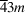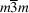International Tables for Crystallography (2006). Vol. A, ch. 1.4, pp. 7-11https://doi.org/10.1107/97809553602060000503

## Contents

• 1.4. Graphical symbols for symmetry elements in one, two and three dimensions  (pp. 7-11)
• 1.4.1. Symmetry planes normal to the plane of projection (three dimensions) and symmetry lines in the plane of the figure (two dimensions)  (p. 7) | html | pdf |
• 1.4.2. Symmetry planes parallel to the plane of projection  (p. 7) | html | pdf |
• 1.4.3. Symmetry planes inclined to the plane of projection (in cubic space groups of classesandonly)  (p. 8) | html | pdf |
• 1.4.4. Notes on graphical symbols of symmetry planes  (p. 8) | html | pdf |
• 1.4.5. Symmetry axes normal to the plane of projection and symmetry points in the plane of the figure  (p. 9) | html | pdf |
• 1.4.6. Symmetry axes parallel to the plane of projection  (p. 10) | html | pdf |
• 1.4.7. Symmetry axes inclined to the plane of projection (in cubic space groups only)  (p. 10) | html | pdf |
• References | html | pdf |